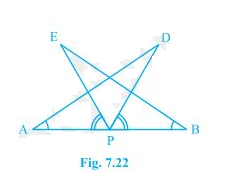# AB is a line segment and P is its mid-point. D and E are points on the same side of AB such that BAD = ABE and EPA = DPB (see Fig.) . Show that (i) ΔDAP ΔEBP (ii) AD = BE#### Solution

Given

P is the mid-point of line segment AB.

BAD = ABE and EPA = DPB

To Prove

(i) ∆ DAP ≅ ∆ EBP

Proof

∠ BAD = ∠ ABE and ∠ EPA = ∠ DPB

On adding ∠DPE on both the sides of two equal angle ∠ EPA = ∠ DPB

∠ EPA + ∠ DPE = ∠ DPB + ∠DPE

∠ DPA = ∠ EPB

From ∆ DAP and ∆ EBP

∠ DPA = ∠ EPB {from above }…… (i)

AP = BP { Given }……………… (ii)

∠ BAD = ∠ ABE { given }…………..(iii)

From above three equation both the triangle satisfies “ASA” congruency criterion

So, ΔDAP ≅ ΔEBP

(ii) AD and BE are equal as they are corresponding parts of congruent triangles(CPCT).

Hence Proved